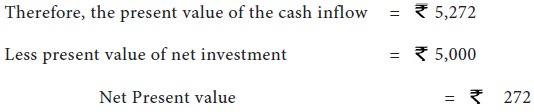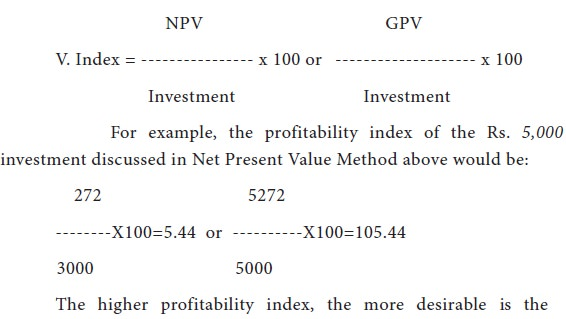Home | ARTS | Financial Management | Discounted Cash flows Techniques - Evaluation Of Capital Projects

# Discounted Cash flows Techniques - Evaluation Of Capital Projects

Posted On :  19.06.2018 11:33 pm

Another method of computing expected rates of return is the present value method.

Discounted Cash flows Techniques

Another method of computing expected rates of return is the present value method. The method is popularly known as Discounted Cash flow Method also. This method involves calculating the present value of the cash benefits discounted at a rate equal to the firm’s cost of capital. In other words, the “present value of an investment is the maximum amount a firm could pay for the opportunity of making the investment without being financially worse off.”

The financial executive compares the present values with the cost of the proposal. If the present value is greater than the net investment, the proposal should be accepted. Conversely, if the present value is smaller than the net investment, the return is less than the cost of financing. Making the investment in this case will cause a financial loss to the firm.

There are four methods to judge the profitability of different proposals on the basis of this technique

## Net Present Value Method

This method is also known as Excess Present Value or Net Gain Method. To implement this approach, we simply find the present value of the expected net cash inflows of an investment discounted at the cost of capital and subtract from it the initial cost outlay of the project. If the net present value is positive, the project should be accepted: if negative, it should be rejected.

NPV = Total Present value of cash inflows – Net Investment

If the two projects are mutually exclusive the one with higher net present value should be chosen. The following example will illustrate the process:

Assume, the cost of capital after taxes of a firm is 6%. Assume further, that the net cash-inflow (after taxes) on a Rs. 5,000 investment is forecasted as being  2,800 per annum for 2 years. The present value of this stream of net cash-inflow discounted at 6% comes to 5,272 (1,813 x   2800).## Internal Rate of Return Method

This method is popularly known as time adjusted rate of return method/discounted rate of return method also. The internal rate of return is defined as the interest rate that equates the present value of expected future receipts to the cost of the investment outlay. This internal rate of return is found by trial and error. First we compute the present value of the cash-flows from an investment, using an arbitrarily elected interest rate. Then we compare the present value so obtained with the investment cost. If the present value is higher than the cost figure, we try a higher rate of interest and go through the procedure again. Conversely, if the present value is lower than the cost, lower the interest rate and repeat the process. The interest rate that brings about this equality is defined as the internal rate of return. This rate of return is compared to the cost of capital and the project having higher difference, if they are mutually exclusive, is adopted and other one is rejected. As the determination of internal rate of return involves a number of attempts to make the present value of earnings equal to the investment, this approach is also called the Trial and Error Method,

## Profitability Index Method

One major disadvantage of the present value method is that it is not easy to rank projects on the basis of net present value particularly when the cost of projects differs significantly. To compare such projects the present value profitability index is prepared. The index establishes relationship between cash-inflows and the amount of investment as per formula given below:investment. Thus, this index provides a ready compatibility of investment having various magnitudes. By computing profitability indices for various projects, the financial manager can rank them in order of their respective rates of profitability.

## Terminal Value Method

This approach separates the timing of the cash-inflows and outflows more distinctly. Behind this approach is the assumption that each cash-inflow is re-invested in other assets at the certain rate of return from the moment, it is received until the termination of the project. Then the present value of the total compounded sum is calculated and it is compared with the initial cash-outflow. The decision rule is that if the present value of the sum total of the compounded re-invested cash-inflows is greater than the present value of cash-outflows, the proposed project is accepted otherwise not. The firm would be different if both the values are equal.

This method has a number of advantages. It incorporates the advantage of re-investment of cash-inflows by compounding and then discounting it. Further, it is best suited to cash budgeting requirements. The major practical problem of this method lies in projecting the future rates of interest at which the intermediate cash inflows received will be re-invested.

## Discounted Cash flow Techniques – Merits

1. This method takes into account the entire economic life of an investment and income there from. It gives the rate of return offered by a new project.

2. It gives due weight to time factor of financing. In the words of Charles Horngreen “Because the discounted cash-flow method explicitly and routinely weights the time value of money, it is the best method to use for long-range decisions.

3. It permits direct comparison of the projected returns on investments with the cost of borrowing money which is not possible in other methods.

4. It makes allowance for differences in the time at which investment generate their income.

5. This approach by recognising the time factor makes sufficient provision for uncertainty and risk. It offers a good measure of relative profitability of capital expenditure by reducing the earnings to the present values.

## Discounted Cash flow Techniques – Demerits

This method is criticized on the following grounds:

1. It involves a good amount of calculations. Hence it is difficult and complicated one. But this criticism has no force.

2. It is very difficult to forecast the economic life of any investment exactly.

3. The selection of cash-inflow is based on sales forecasts which are in itself an indeterminable element.

4. The selection of an appropriate rate of interest is also difficult.
Tags : Financial Management - Capital Budgeting – A Conceptual Framework
Last 30 days 139 views